﻿ 弹性支撑圆柱绕流稳定性分析
«上一篇文章快速检索 高级检索

 力学学报2015, Vol. 47Issue (5): 874-880  DOI: 10.6052/0459-1879-14-3070

### 引用本文 [复制中英文]

[复制中文]
Li Xintao, Zhang Weiwei, Jiang Yuewen, Ye Zhengyin. STABILITY ANALYSIS OF FLOW PAST AN ELASTICALLY-SUSPENDED CIRCULAR CYLINDER[J]. Chinese Journal of Ship Research, 2015, 47(5): 874-880. DOI: 10.6052/0459-1879-14-307.
[复制英文]

### 文章历史

2014-10-10 收稿
2015-06-06 录用
2015–06–10 网络版发表

CFD技术已经广泛应用于流固耦合力学问题的研究. 然而基于CFD技术的流固耦合直接数值模拟的计算花费仍然很大，不适合参 数的分析和系统的设计. 近年来研究者纷纷寻求高效、高精度的非定常气动力模型以适应流固耦合力学的发展.

1 非定常气动力计算与建模

 $y(k) = \sum\limits_{i = 1}^{na} {{A_i}y(k - i)} + \sum\limits_{i = 0}^{nb - 1} {{B_i}u(k - i) + e(k)}$ (1)

 ${ x }_{ a } (k) = [{ y }_{ a } (k - 1), \cdots { y }_{ a } (k - na),\\ \qquad { u }(k - 1),\cdots ,{ u }(k - nb + 1)]^{\rm T}$ (2)

 $\left. \begin{array}{l} {x_a}(k + 1) = {{\tilde A}_a}{x_a}(k) + {{\tilde B}_a}u(k)\\ {y_a}(k) = {{\tilde C}_a}{x_a}(k) + {{\tilde D}_a}u(k) \end{array} \right\}$ (3)

 $\begin{array}{l} {{\tilde A}_a} = \left[{\begin{array}{*{20}{c}} {{A_1}}&{{A_2}}& \cdots &{{A_{na - 1}}}&{{A_{na}}}&{{B_1}}&{{B_2}}& \cdots &{{B_{nb - 2}}}&{{B_{nb - 1}}}\\ I&0& \cdots &0&0&0&0& \cdots &0&0\\ \vdots &I& \cdots &0&0&0&0& \cdots &0&0\\ \vdots & \vdots & \ddots & \vdots & \vdots & \vdots & \vdots & \ddots & \vdots & \vdots \\ 0&0& \cdots &I&0&0&0& \cdots &0&0\\ 0&0& \cdots &0&0&0&0& \cdots &0&0\\ 0&0& \cdots &0&0&I&0& \cdots &0&0\\ 0&0& \cdots &0&0&0&I& \cdots &0&0\\ \vdots & \vdots & \ddots & \vdots & \vdots & \vdots & \vdots & \ddots & \vdots & \vdots \\ 0&0& \cdots &0&0&0&0& \cdots &I&0 \end{array}} \right]\\ {{\tilde B}_a} = {\left[{\begin{array}{*{20}{c}} {{{\tilde B}_0}\;\;0\;\;0\;\; \cdots \;\;0\;\;I\;\;0\;\;0\;\; \cdots \;\;0} \end{array}} \right]^{\rm{T}}}\\ {{\tilde C}_a} = \left[{\begin{array}{*{20}{c}} {{A_1}\;\;{A_2}\;\; \cdots \;\;{A_{na - 1}}\;\;{A_{na}}\;\;{B_1}\;\;{B_2}\;\; \cdots \;\;{B_{nb - 2}}\;\;{B_{nb - 1}}} \end{array}} \right]\\ {D_a} = \left[{{B_0}} \right] \end{array}$

 $\left.\!\!\begin{array}{l} \dot{ x }_{ a} (t) = { A }_{ a} { x }_{ a } (t) + { B }_{ a } { u} (t) \\ { y }_{ a } (t) = { C }_{ a } { x}(t) + { D }_{ a } { u }(t) \end{array} \!\! \right \}$ (4)

2 基于ROM的弹性支撑圆柱绕流分析模型

 ${ M } \cdot \ddot { \xi } + { G } \cdot \dot { \xi } + { K } \cdot { \xi } = { Q}$ (5)

 ${ M } = \left[\!\! \begin{array}{cc} 1 & 0 \\ 0 & 1 \end{array}\!\! \right]\,,\ \ { K }=\left[\!\! \begin{array}{cc} {(2\pi F_n )^2} & 0 \\ 0 & {(2\pi F_n )^2} \end{array}\!\! \right] \\ { G}=\left\{\!\!\begin{array}{cc} {4\pi F_n \zeta } & 0 \\ 0 & {4\pi F_n \zeta } \end{array}\!\!\right\}\,,\ \ { Q }=q\left\{ \!\! \begin{array}{c} {C_L } \\ {8C_m } \end{array} \!\! \right\}\,,\ \ q = \dfrac{2}{\pi M}$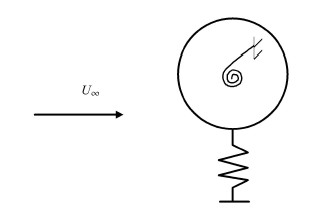图 1 两自由度振荡圆柱 Fig. 1 Oscillating cylinder with two degrees of freedom

$F_n = f_n D / U_\infty$为圆柱的无量纲非耦合固有频率，其中 $f_n$为结构固有频率，$D$为圆柱直径，$U_\infty$为来流速度. $\zeta$为结构阻尼系数，在本文中设定为0. $M = 4m / (\pi \rho D^2)$为质量比，$m$和 $\rho$分别为圆柱的质量和来流的密度，圆柱的位移和速度分别用 $D$和 $U_\infty$ 进行无量纲化. 特别地，定义 $U^ * = 1 / F_n = U_\infty / (f_n D)$为折减速度.

 $\left.\!\!\begin{array}{l} \dot{ x }_{ s } (t) = { A }_{ s } { x}_{s} (t) + q{ B}_{s} { y}_{a} (t) \\ \dot {\xi }(t) = { C}_{s} { x}_{s} (t) + q{ D}_{s} { y}_{a} (t) \end{array} \!\! \right\}$ (6)

 ${ A}_{s}= \left[\!\!\begin{array}{cc} {\bf 0} & { I} \\ -{ M}^{- 1}{ K} & -{ M}^{- 1}{ G} \end{array} \!\! \right]\,, \quad { B}_{s} = \left[\!\!\begin{array}{c} {\bf 0} \\ { M}^{ -1} \end{array} \!\! \right] \\ { C}_{s} = \left[\!\!\begin{array}{cc} { I} & {\bf 0} \end{array}\!\!\right]\,, \quad { D}_{s} = {\bf 0}$

 $\left. \begin{array}{l} {{\dot x}_{as}}(t) = \left[ {\begin{array}{*{20}{c}} {{A_s} + q{B_s}{D_s}{C_s}}&{amp;q{B_s}{C_a}}\\ {{B_a}{C_s}}&{amp;{A_a}} \end{array}} \right]{x_{as}}(t)\\ \xi (t) = \left[ {\begin{array}{*{20}{c}} {{C_s}}&{amp;{\bf{0}}} \end{array}} \right]{x_{as}}(t) \end{array} \right\}$ (7)

3 算例与分析 3.1 非定常气动力建模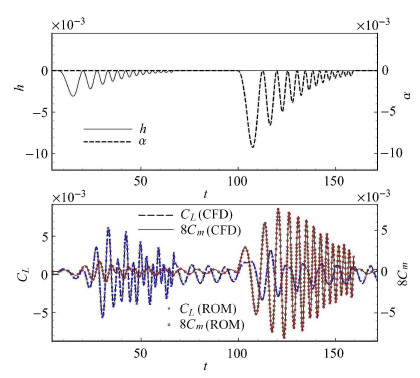图 2 2= 45, 扫频信号输入下CFD 结果和辨识模型解的比较 Fig. 2 Comparison of CFD solutions and the identified model solutions for the chirp input signal at Re = 45
3.2 单自由度横向振荡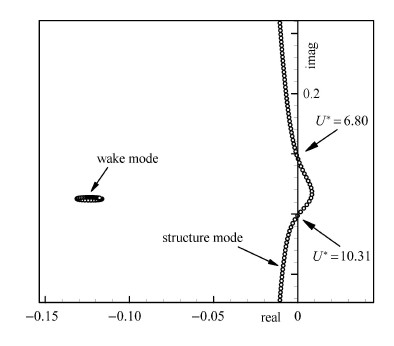图 3 Re = 33, M = 50 时弹性系统的根轨迹图 Fig. 3 Root loci of the coupled system for Re = 33, M = 50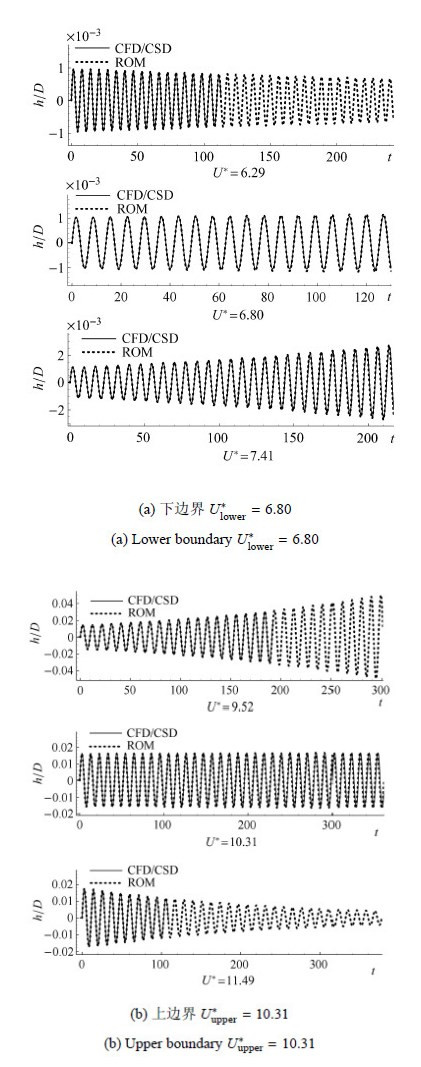图 4 Re = 33, M = 50 临界响应对比 Fig. 4 Comparasions of critical time responses for Re = 33, M = 50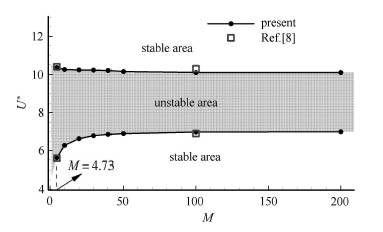图 5 稳定性边界随质量比的变化 Fig. 5 Instability boundaries versus the mass ratio

$Re$对流动和流固耦合系统的动力学特征具有重要的影响. 在这里固定 $M = 50$，研究$Re$的影响. 图6给出了不同$Re$下弹性系统的根轨迹图. 图7给出了稳定性边界随$Re$的变化的稳定性包线. 从图中可以看出在 $Re = 20$时，系统是临界稳定的；当$Re < 20$时，在任何结构固有频率下弹性系统都不会出现失稳，这与Mittal等通过大量数值计算分析的结果一致. 而本文只需要通过几个典型$Re$的信号训练，得到相应$Re$下的降阶气动力模型，然后耦合结构运动方程，得到弹性系统 的降阶模型，通过特征分析便很快能够得到如图7所示的稳定性包线.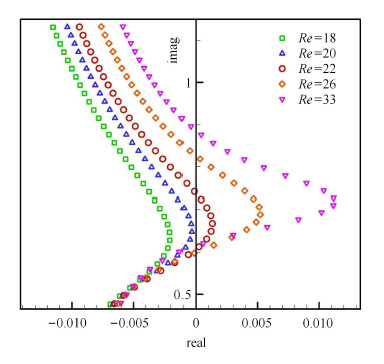图 6 不同Re下弹性系统的根轨迹图 Fig. 6 Root loci of the coupled system for different Re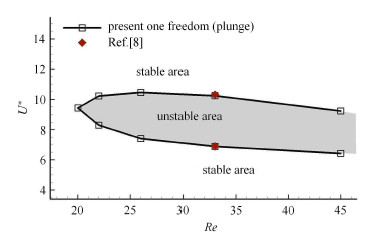图 7 稳定性边界随Re的变化 Fig. 7 Instability boundaries varying with Re
3.3 旋转自由度释放对弹性系统稳定性的影响

3.2节分析表明，单自由度横向支撑圆柱的失稳临界$Re_{\rm cr}$约为20. 一个很有趣的问题是，弹性支撑圆柱绕流的临界$Re_{\rm cr}$是否能够进一步降低，多自由度释放对弹性系统的稳定性有无影响. 这里同样固定质量比$M =50$, 研究旋转自由度释放对弹性系统稳定性的影响. 图8给出了不同雷诺数下，单自由度旋转支撑圆柱的特征值随$F_n$变化的根轨迹图. 从图中看出各状态下弹性系统都是稳定的，说明单独旋转自由度释放不会影响系统的稳定性，即不会降低 系统的临界雷诺数.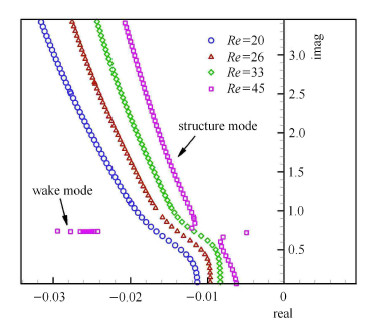图 8 单自由旋转支撑圆柱的根轨迹图 Fig. 8 Root loci of one freedom pitching system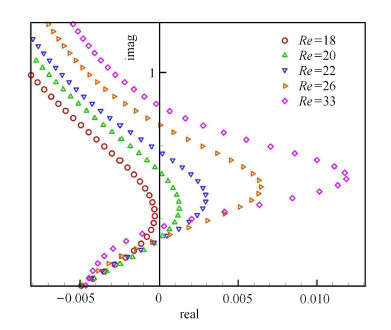图 9 二自由系统的根轨迹图 Fig. 9 Root loci of two freedom coupled system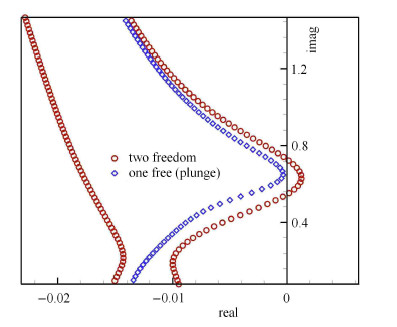图 10 Re = 20 时不同支撑方式根轨迹图对比 Fig. 10 Comparison of root loci for Re = 20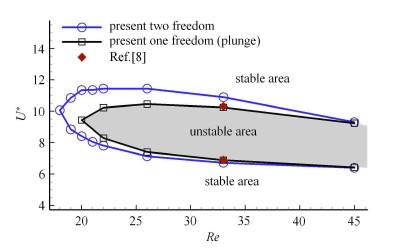图 11 稳定性边界对比 Fig. 11 Comparison of instability boundaries
4 结 论

(1)本文采用系统辨识方法首次建立了绕圆柱流动的非定常气动力模型. 该模型是一个线性动力学模型，能够准确捕获流动的稳定性特征. 这一工作为将来的圆柱绕流主动控制研究奠定了基础.

(2)大部分的涡致振动研究集中在圆柱单自由度横向振动的情形，很少有研究者关注旋转自由度释放对耦合系统的影响. 本文首次研究了旋转自由度释放对系统稳定性特征的影响. 研究表明，相对于单自由度横向支撑方式，旋转自由度的释放能够进一步降低系统的稳定性.

(3) ROM不仅具有很高的计算效率，而且能够深刻揭示弹性支撑圆柱绕流失稳的根本原因：流动模态和结构模态耦合作用导致结构模态失稳所致.

  Williamson CHK. Vortex dynamics in the cylinder wake. Annual Review of Fluid Mechanics, 1996, 28(1): 477-539  陈文曲, 任安禄, 李广望. 串列双圆柱绕流下游圆柱两自由度涡致振动研究. 力学学报, 2004, 36(6): 732-738 (Chen Wenqu, Ren Anlu, Li Guangwang. The numerical study of two-degree of freedom vortex-induced vibrations of the downstream cylinder in tandem arrangement. Chinese Journal of Theoretical and Applied Mechanics, 2004, 36(6): 732-738 (in Chinese))  宋芳, 林黎明, 凌国灿. 圆柱涡激振动的结构-尾流振子耦合模型研究. 力学学报, 2010, 42(3): 357-365 (Song Fang, Lin Liming, Lin Guocan. The study of vortex-induced vibrations by computation using coupling model of structure and wake oscillator. Chinese Journal of Theoretical and Applied Mechanics, 2010, 42(3): 357-365 (in Chinese))  王俊高, 付世晓, 许玉旺等. 正弦振荡来流下柔性立管涡激振动发展过程. 力学学报, 2014, 46(2): 173-182 (Wang Jungao, Fu shixiao, Xu yuwang, et al. VIV development process of a flexible cylinder under oscillatory flow. Chinese Journal of Theoretical and Applied Mechanics, 2014, 46(2): 173-182 (in Chinese))  Bearman PW. Circular cylinder wakes and vortex-induced vibrations. Journal of Fluids and Structures, 2011, 27(5): 648-658  Williamson CHK, Govardhan R. Vortex-induced vibrations. Annual Review of Fluid Mechanics, 2004, 36: 413-455  Cossu C, Morino L. On the instability of a spring-mounted circular cylinder in a viscous flow at low Reynolds numbers. Journal of Fluids and Structures, 2000, 14(2): 183-196  Mittal S, Singh S. Vortex-induced vibrations at subcritical Re. Journal of Fluid Mechanics, 2005, 534: 185-194  张伟伟, 叶正寅. 基于非定常气动力辨识技术的气动弹性数值模拟. 航空学报, 2006, 27(4): 579-583 (Zhang Weiwei, Ye Zhengyin. Numerical simulation of aeroelasticity basing on identification technology of unsteady aerodynamic loads. Acta Aeronautica et Astronautica Sinica, 2006, 27(4): 579-583 (in Chinese))  张伟伟, 叶正寅, 杨青. 基于 ROM 技术的阵风响应分析方法. 力学学报, 2008, 40(5): 593-598 (Zhang Weiwei, Ye Zhengyin, Yang Qing. Gust response analysis using CFD-based reduced order models. Chinese Journal of Theoretical and Applied Mechanics, 2008, 40(5): 593-598 (in Chinese))  Cowan TJ, Arena AS, Gupta KK. Accelerating computational fluid dynamics based aeroelastic predictions using system identification. Journal of Aircraft, 2001, 38(1): 81-87  蒋跃文. 基于广义网格的CFD方法及其应用. [博士论文]. 西安:西北工业大学, 2013 (Jiang Yuewen. Numerical solution of Navier-Stokers equations on generalized mesh and its applications. [PhD Thesis]. Xi'an: Northwestern Polytechnical University, 2013 (in Chinese))
STABILITY ANALYSIS OF FLOW PAST AN ELASTICALLY-SUSPENDED CIRCULAR CYLINDER
Li Xintao, Zhang Weiwei, Jiang Yuewen, Ye Zhengyin
National Key Laboratory of Aerodynamic Design and Research, Northwestern Polytechnical University, Xi'an 710072, China
Fund: The project was supported by the National Natural Science Foundation of China (11072199, 11172237), Program for New Century Excellent Talents in University (NCET-13-0478) and the Youth Nova of Science and Technology of Shanxi Province.
Abstract: An identification technique is used to construct reduced order models (ROMs) for the cylinder wake flow at subcritical Reynolds numbers (Re <47) using CFD simulation data. ROM-based aeroelastic models are then found by coupling the structural motion equations and ROMs. The impacts of structural frequency, mass ratio and mount style on the instability boundaries are investigated. It is found that self-excited oscillations, accompanied by vortex shedding are possible at Re as low as 20 for one freedom transversely suspended cylinder, as the structural frequency gets close to that of the most unstable mode of flow. The release of rotational freedom could further increase the instability of the coupled system and the critical Re can be reduced to 18. ROM-based method not only has high efficiency but also profoundly shows that the inherent instability of the coupled system is induced by the unstable of the structure mode. Therefore, in the unstable region, the vibration frequency of the coupled system is synchronized with the natural frequency of the cylinder.
Key words: cylinder    system identification    ROM    vortex induced vibration    stability analysis